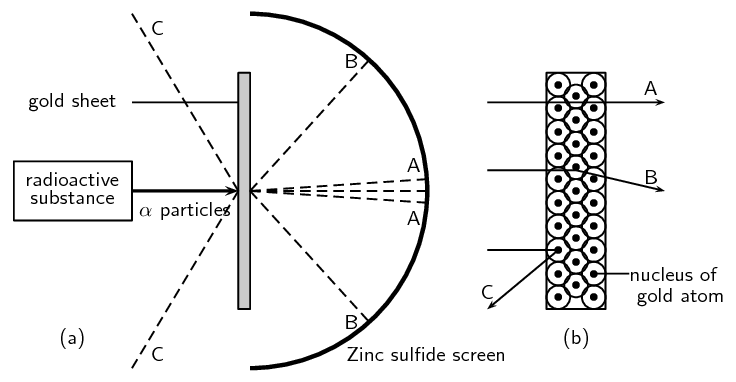We think you are located in United States. Is this correct?

# 4.3 Atomic mass and diameter

## 4.3 Atomic mass and diameter (ESAAU)

It is difficult sometimes to imagine the size of an atom, or its mass, because we cannot see an atom and also because we are not used to working with such small measurements.

### How heavy is an atom? (ESAAV)

It is possible to determine the mass of a single atom in kilograms. But to do this, you would need special instruments and the values you would get would be very clumsy and difficult to work with. The mass of a carbon atom, for example, is about $$\text{1,99} \times \text{10}^{-\text{26}}$$ $$\text{kg}$$, while the mass of an atom of hydrogen is about $$\text{1,67} \times \text{10}^{-\text{27}}$$ $$\text{kg}$$. Looking at these very small numbers makes it difficult to compare how much bigger the mass of one atom is when compared to another.

To make the situation simpler, scientists use a different unit of mass when they are describing the mass of an atom. This unit is called the atomic mass unit ($$\text{amu}$$). We can abbreviate (shorten) this unit to just $$\text{u}$$. Scientists use the carbon standard to determine $$\text{amu}$$. The carbon standard gives carbon an atomic mass of $$\text{12,0}$$ $$\text{u}$$. Compared to carbon the mass of a hydrogen atom will be $$\text{1}$$ $$\text{u}$$. Atomic mass units are therefore not giving us the actual mass of an atom, but rather its mass relative to the mass of one (carefully chosen) atom in the periodic table. In other words it is only a number in comparison to another number. The atomic masses of some elements are shown in Table 4.1.

 Element Atomic mass ($$\text{u}$$) Carbon ($$\text{C}$$) $$\text{12,0}$$ Nitrogen ($$\text{N}$$) $$\text{14,0}$$ Bromine ($$\text{Br}$$) $$\text{79,9}$$ Magnesium ($$\text{Mg}$$) $$\text{24,3}$$ Potassium ($$\text{K}$$) $$\text{39,1}$$ Calcium ($$\text{Ca}$$) $$\text{40,1}$$ Oxygen ($$\text{O}$$) $$\text{16,0}$$

Table 4.1: The atomic mass number of some of the elements.

The actual value of 1 atomic mass unit is $$\text{1,66} \times \text{10}^{-\text{24}}$$ $$\text{g}$$ or $$\text{1,66} \times \text{10}^{-\text{27}}$$ $$\text{kg}$$. This is a very tiny mass! If we write it out it looks like this: $$\text{0,00000000000000000000000000166}$$ $$\text{kg}$$. An atom is therefore very very small.

### Rutherford's alpha-particle scattering experiment (ESAAW)

Radioactive elements emit different types of particles. Some of these are positively charged alpha (α) particles. Rutherford wanted to find out where the positive charge in an atom is. He carried out a series of experiments where he bombarded sheets of gold foil with alpha particles (since these would be repelled by the positive nucleus). A simplified diagram of his experiment is shown in Figure 4.6.Figure 4.6: Rutherford's gold foil experiment. Figure (a) shows the path of the α particles after they hit the gold sheet. Figure (b) shows the arrangement of atoms in the gold sheets and the path of the α particles in relation to this.

Rutherford set up his experiment so that a beam of alpha particles was directed at the gold sheets. Behind the gold sheets was a screen made of zinc sulfide. This screen allowed Rutherford to see where the alpha particles were landing. Rutherford knew that the electrons in the gold atoms would not really affect the path of the alpha particles, because the mass of an electron is so much smaller than that of a proton. He reasoned that the positively charged protons would be the ones to repel the positively charged alpha particles and alter their path.

If Thomson's model of the atom was correct then Rutherford would have observed mostly path A in Figure 4.6. (A represents alpha particles that pass straight through the positive nucleus.) He would not have observed any deflection of the alpha particles. What he found instead was that while most of the alpha particles passed through the foil undisturbed and could be detected on the screen directly behind the foil (path A), some of the particles ended up being slightly deflected onto other parts of the screen (path B)! The fact that some particles were deflected suggested that the positive charge was concentrated in one part of the atom only.

Through this experiment he concluded that the nucleus of the atom is positively charged and situated in the middle of the atom.

### How small is the nucleus? (ESAAX)

The nucleus of an atom is very small. We can compare an atom with a soccer stadium. If the whole atom is as big as a soccer stadium, the nucleus is only the size of a pea in the middle of the stadium! This should help you see that the atom is mainly made up of empty space. If you removed all the empty space from the atoms in your body, you would become the size of a grain of salt!

### Relative atomic mass (ESAAY)

Relative atomic mass

The relative atomic mass of an element is the average mass of all the naturally occurring isotopes of that element. The units for relative atomic mass are atomic mass units.

The relative atomic mass of an element is the number you will find on the periodic table.

temp text